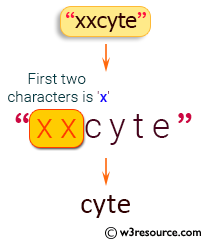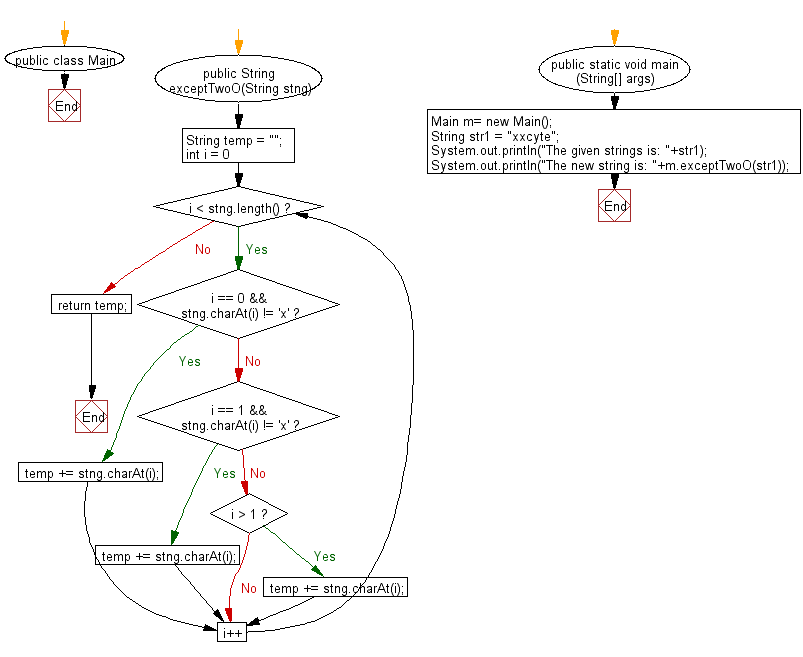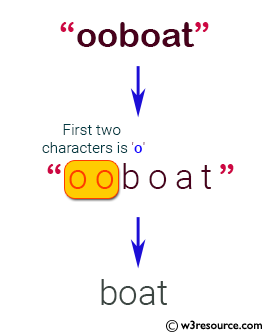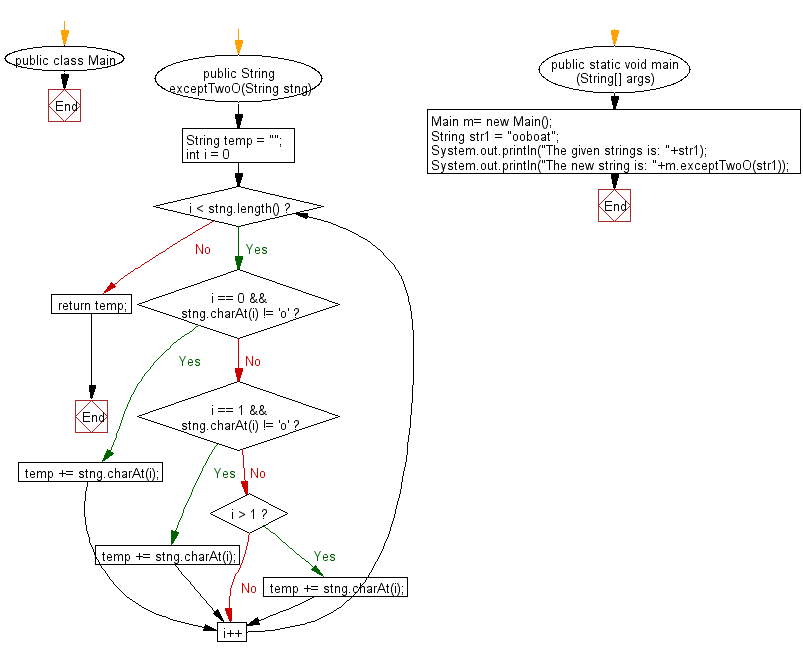﻿ Java exercises: Read a string and if one or both of the first two characters is 'x', return without those 'x', otherwise return the string unchanged - w3resource# Java String Exercises: Read a string and if one or both of the first two characters is 'x', return without those 'x', otherwise return the string unchanged

## Java String: Exercise-67 with Solution

Write a Java program to read a string and if one or both of the first two characters is 'x', return without those 'x',otherwise return the string unchanged.

Sample Solution:

Java Code:

``````import java.util.*;
public class Main
{
public String exceptTwoO(String stng)
{
String temp = "";
for (int i = 0; i < stng.length(); i++)
{
if (i == 0 && stng.charAt(i) != 'x')
temp += stng.charAt(i);
else if (i == 1 && stng.charAt(i) != 'x')
temp += stng.charAt(i);
else if (i > 1)
temp += stng.charAt(i);
}
return temp;
}
public static void main (String[] args)
{
Main m= new Main();
String str1 =  "xxcyte";
System.out.println("The given strings is: "+str1);
System.out.println("The new string is: "+m.exceptTwoO(str1));
}
}
```
```

Sample Output:

```The given strings is: xxcyte
The new string is: cyte
```

Pictorial Presentation:Flowchart:Java Code:

``````import java.util.*;
public class Main
{
public String exceptTwoO(String stng)
{
String temp = "";
for (int i = 0; i < stng.length(); i++)
{
if (i == 0 && stng.charAt(i) != 'o')
temp += stng.charAt(i);
else if (i == 1 && stng.charAt(i) != 'o')
temp += stng.charAt(i);
else if (i > 1)
temp += stng.charAt(i);
}
return temp;
}
public static void main (String[] args)
{
Main m= new Main();
String str1 =  "ooboat";
System.out.println("The given strings is: "+str1);
System.out.println("The new string is: "+m.exceptTwoO(str1));
}
}
```
```

Sample Output:

```The given strings is: ooboat
The new string is: boat
```

Pictorial Presentation:Flowchart:Java Code Editor:

Improve this sample solution and post your code through Disqus

What is the difficulty level of this exercise?

﻿